# Simultaneous Linear Equations

Go back to  'Linear and Quadratic Equations'

## Introduction to Simultaneous Linear Equations

Sometimes, one is just not enough, and we need two variables to get the job done. That is when simultaneous linear equations come into play. Imagine this situation, 2 bars of Cadbury chocolate and 3 Mars bars cost Rs.270. At the same store, someone else buys 4 bars of Cadbury chocolate and 2 Mars bars and pays Rs.300. What is the cost of one bar of Cadbury chocolate and one Mars bar? These are the types of problems that require you to solve for 2 variables and therefore the need arises for simultaneous equations.

The training wheels are off from this point on and it’s time to dive into the deep end of the middle school math pool.

## The Big Idea: What are Simultaneous Linear Equations?

A system of two equations with the same variables are called simultaneous linear equations. The important thing to remember is that there are 2 unknowns (or variables) and 2 equations (which are linear) in this system. The aim here is to get the pair of values of the variables which satisfy both the equations. For example, both the following equations are linear equations in two variables: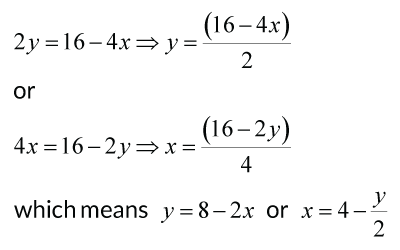As you can see, if we treat these equations with two variables as two independent entities then we can find an infinite number of solutions for both $${x}$$ and $${y}$$. That is not helpful at all! Therefore, we write them as a system of simultaneous equations. That means that the variables are the same and can have unique solutions for both x and y, for example: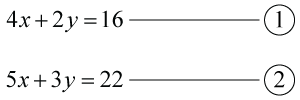Now, we want to find the pair $$(x, y)$$ that satisfies both the equations at the same time. Basically, what that means is that for all the infinite solutions to equation 1, there will be a pair $$(x, y)$$ that also satisfies equation 2. That is what we are looking for. We can visualize a system of simultaneous linear equations by drawing 2 linear graphs and finding out their intersection point.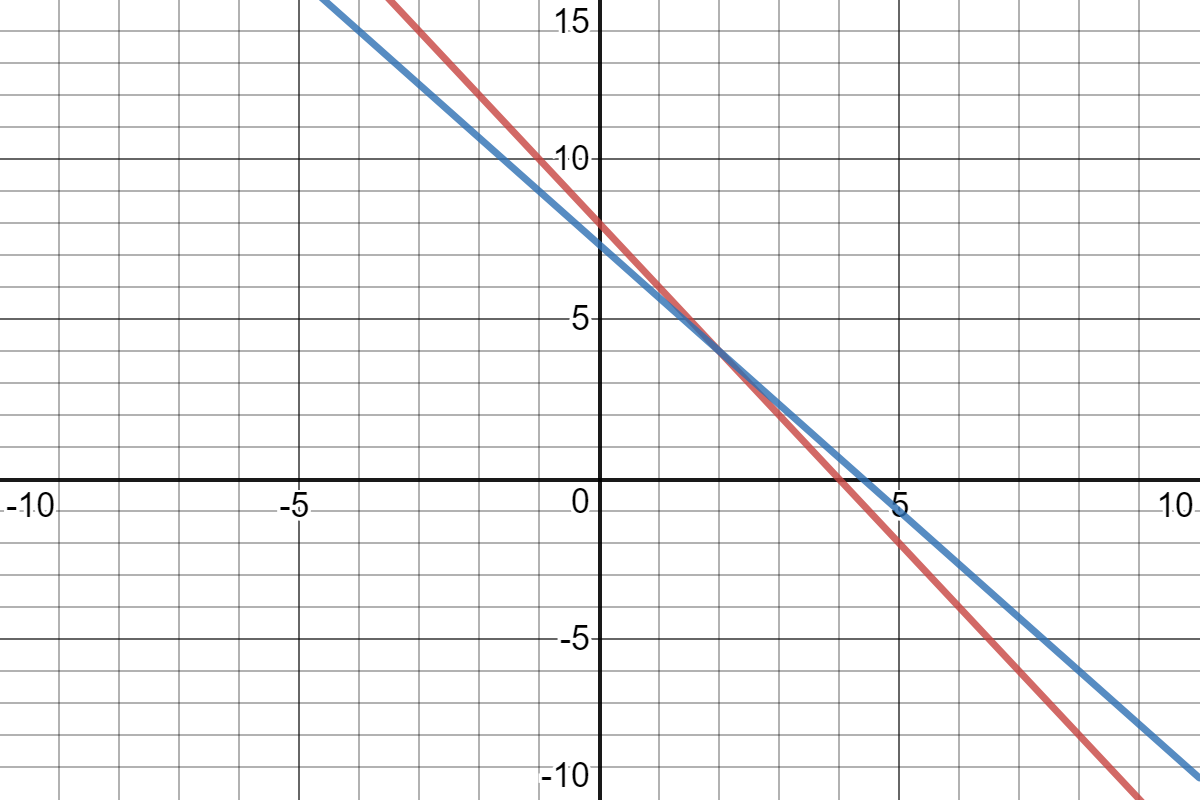The red line represents all the solutions for equation 1, and the blue line, solutions for equation 2. The intersection is the unique $$(x, y)$$ that we are looking for which will satisfy both the equations.

## How is it important?

### How do I solve simultaneous linear equations?

There are three major methods to solve simultaneous linear equations:

• Graphically

• Substitution

• Elimination

While we suggest you start off with the graphical method for simple equations, as it allows for you to visualize the process (as you clearly would have observed in the case of the above widget), it is limiting and hence the other two more abstract methods are more robust and powerful.

Take a look at the example below to understand the Substitution method: you can observe how the values of the variables are obtained by substituting the variable in one equation by its solution of the other equation. The key is to represent one variable as an expression of the other variable.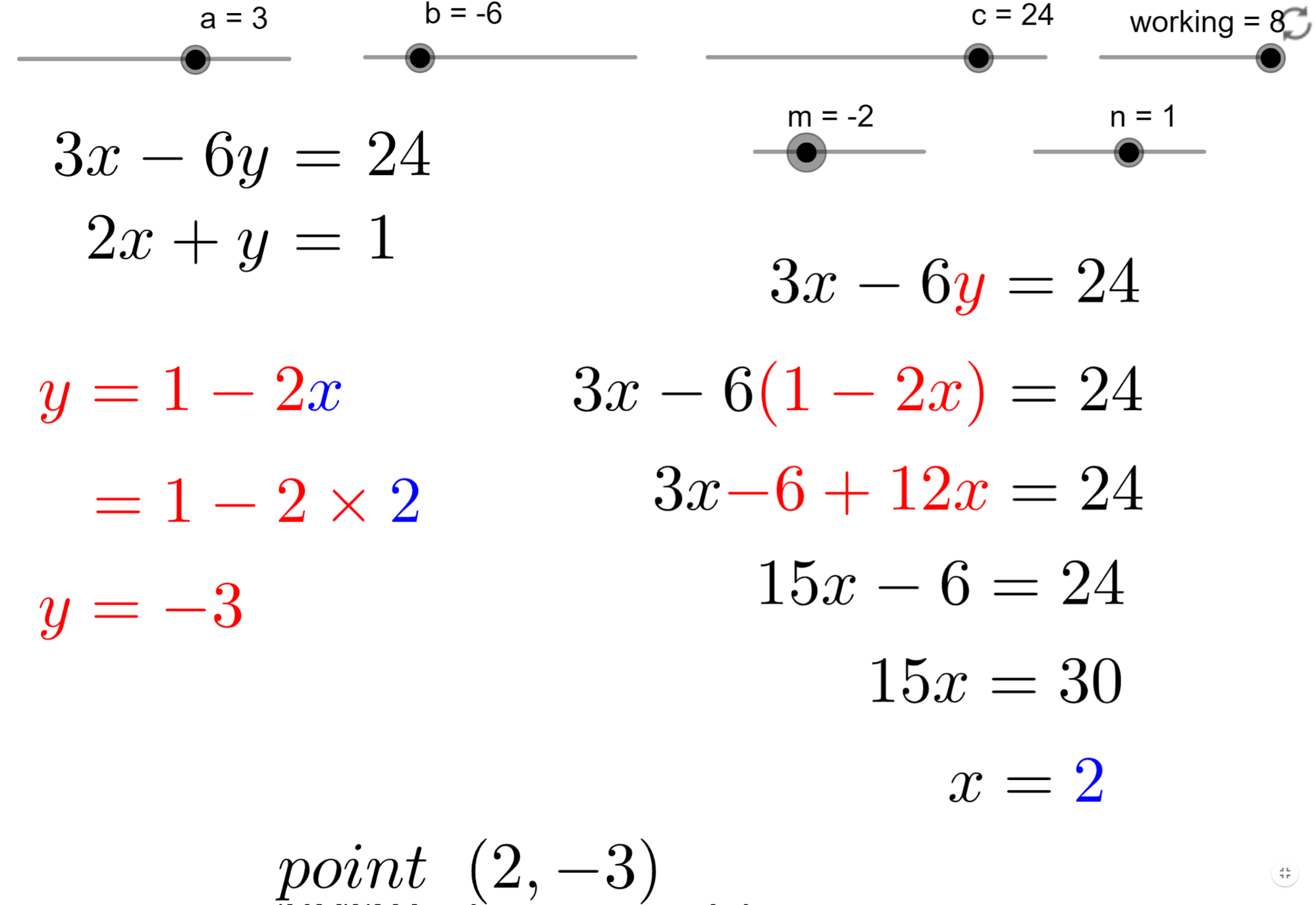Take a look at the image below to understand the Elimination method: In this method we can eliminate one variable by multiplying each of the equations so that when one equation is subtracted from the other, the resulting equation contains only one variable as the coefficient of the other variable reduces to zero.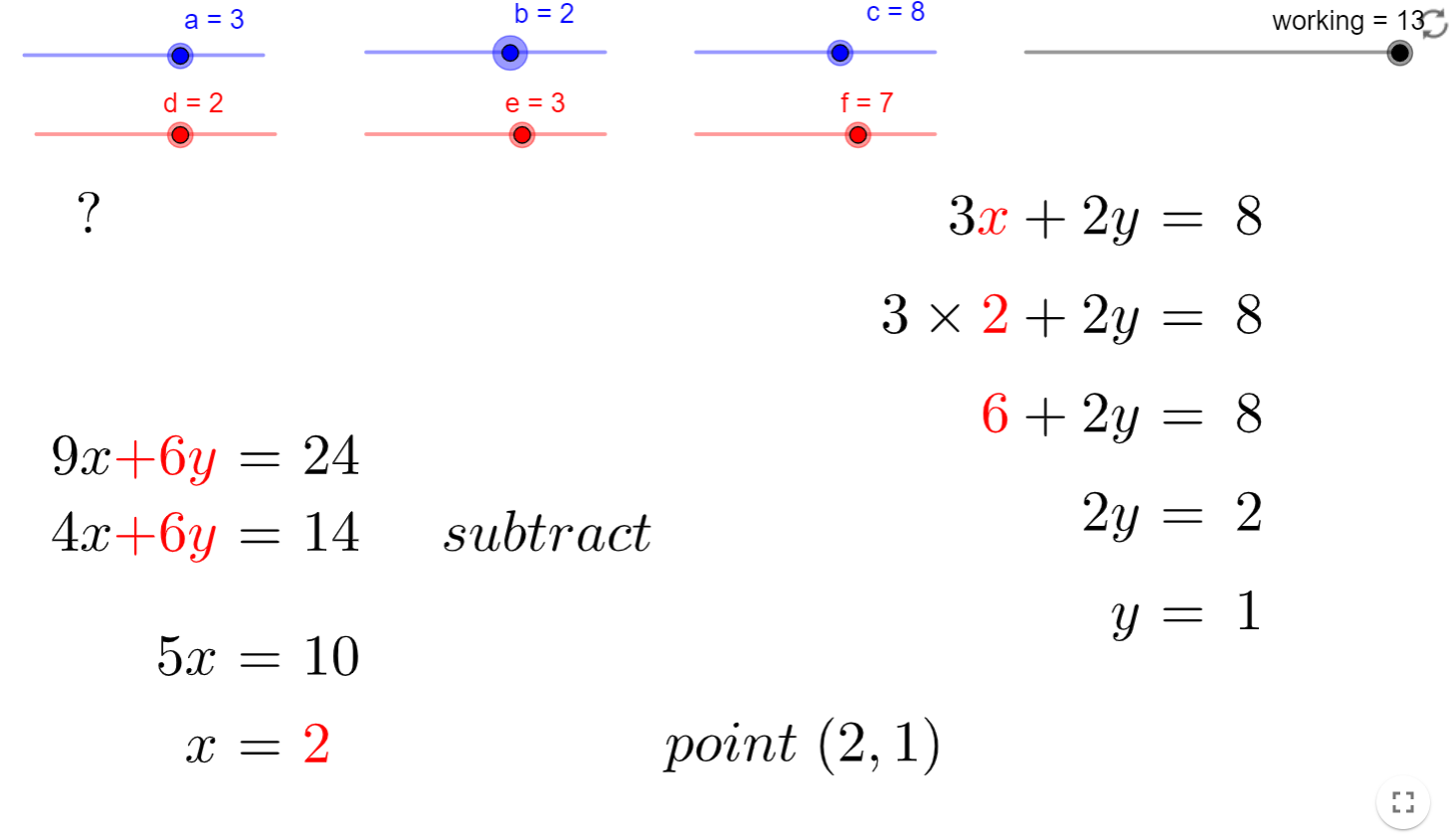Some useful tips to help you speed up the process of solving simultaneous linear equations:

• You can use the graphical, substitution or elimination methods to solve a system of linear equations.

• Simultaneous linear equations may have a unique solution if they intersect at one point• Infinite solutions if the two lines are overlapping: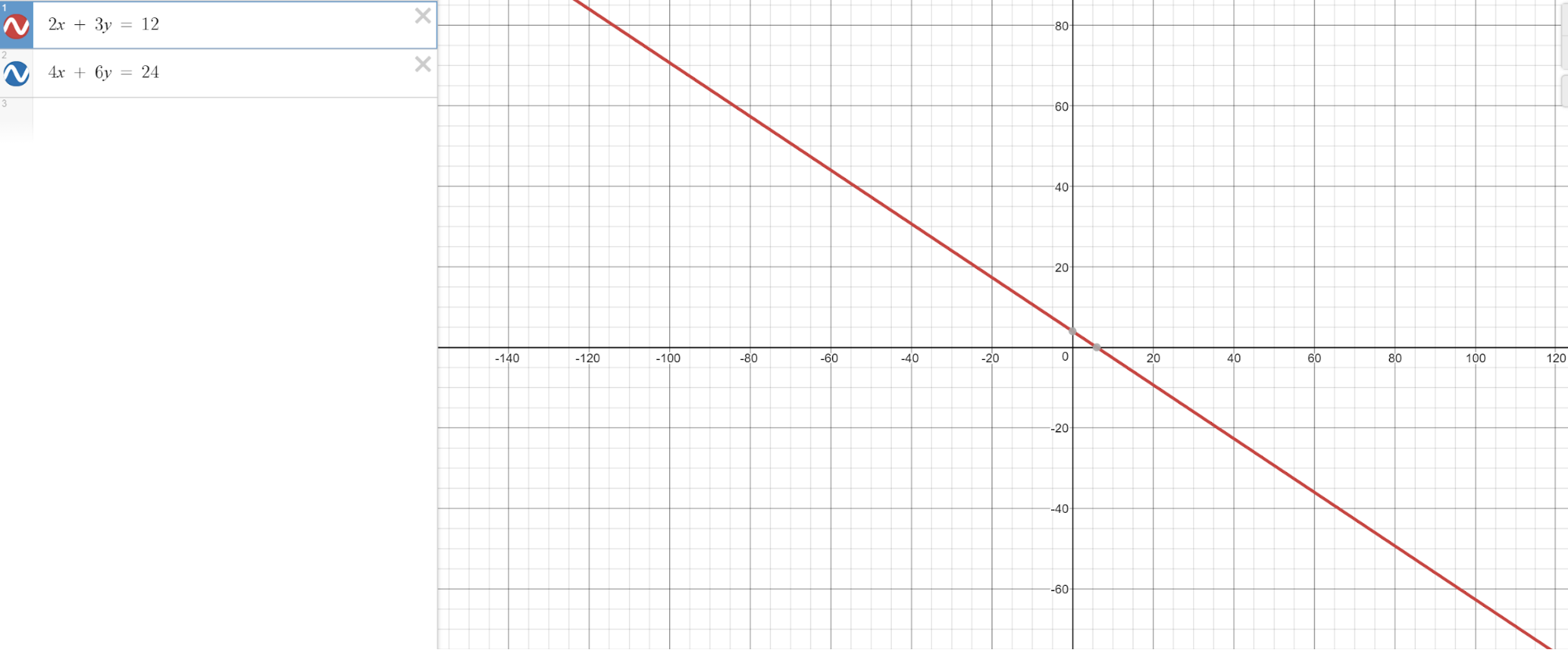• No solutions if the two lines are parallel: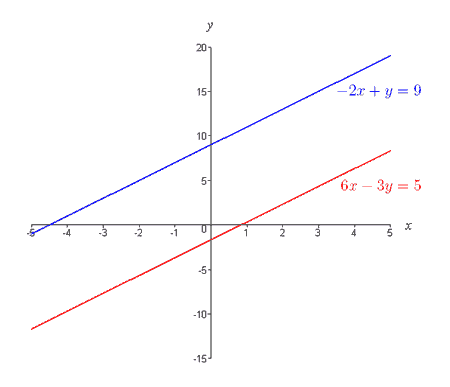• One Unique solution is obtained if the 2 lines intersect at one particular point: this will give an ordered pair $$(x, y)$$ of solution. If the 2 lines lie on top of each other, it is simple to see that the 2 equations are just multiples of one another and they will have an infinite number of ordered pairs $$(x, y)$$ as their solution. In some cases, you may find that the 2 equations actually represent a set of parallel lines; this will mean that the system has no solutions as the 2 lines will never intersect one another.

• It is always recommended that you should substitute the ordered pair of values $$(x, y)$$ back into the simultaneous linear equations to check whether it is indeed the correct solution.